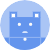search
Search
menu search toc more_vert
Guest 0reps
Thanks for the thanks!
close
chevron_left Data Indexing and Masks
Cancel
Post
account_circle
Profile
exit_to_app
Sign out
help Ask a question
search
keyboard_voice
close
Searching Tips
Search for a recipe:
"Creating a table in MySQL"
Search for an API documentation: "@append"
Search for code: "!dataframe"
Apply a tag filter: "#python"
Useful Shortcuts
/ to open search panel
Esc to close search panel
to navigate between search results
d to clear all current filters
Enter to expand content previewDoc SearchCode Search BetaSORRY NOTHING FOUND!
mic
Start speaking...Voice search is only supported in Safari and Chrome.
Shrink
Navigate to
A
A
brightness_medium
share
arrow_backShareTwitterFacebook
chevron_left Data Indexing and Masks
1
thumb_down
0
chat_bubble_outline
0
auto_stories new
settings

# Pandas DataFrame | loc property

Pandas
chevron_right
Documentation
chevron_right
DataFrame
chevron_right
Data Indexing and Masks
schedule Jul 1, 2022
Last updated
local_offer PythonPandas
Tags

Pandas `DataFrame.loc` is used to access or update values of the DataFrame using row and column labels. Note that `loc` is a property and not a function - we provide the parameters using `[]` notation.

The allowed inputs are as follows:

• numbers and strings (e.g. `3`, `"a"`).

• a list of labels (e.g. `[3,"a"]`).

• slice object (e.g. `"a":"d"`). Unlike standard Python slices, both ends are inclusive.

• a boolean array where rows/columns corresponding to `True` will be returned.

• a function that takes as input the source DataFrame and returns one of the above.

NOTE

Numbers are treated as labels, and so they are always casted to a string.

# Return Value

If the result is a single row or column, then a `Series` is returned. Otherwise, a `DataFrame` is returned.

# Examples

## Accessing a single value

Consider the following DataFrame:

``` df = pd.DataFrame({"A":[3,4],"B":[5,6]}, index=["a","b"])df    A  Ba  3  5b  4  6 ```

To access the value at `[bB]` using row and column labels:

``` df.loc["b","B"] 6 ```

## Accessing rows

Consider the following DataFrame:

``` df = pd.DataFrame({"A":[3,4,5],"B":[6,7,8]}, index=["a","b","c"])df    A  Ba  3  6b  4  7c  5  8 ```

### Access a single row

To get row `b`:

``` df.loc["b"] A 4B 7Name: b, dtype: int64 ```

### Access multiple rows

To access multiple rows, pass in a list of row labels like so:

``` df.loc[["a","c"]]    A  Ba  3  6c  5  8 ```

You could also use slicing syntax like so:

``` df.loc["a":"b"]    A  Ba  3  6b  4  7 ```

Notice, unlike Python's standard slicing behavior, both ends are inclusive.

## Accessing columns

Consider the following DataFrame:

``` df = pd.DataFrame({"A":[3,4],"B":[5,6]}, index=["a","b"])df    A  Ba  3  5b  4  6 ```

### Accessing a single column

To access a single column:

``` df.loc[:,"A"] a 3b 4Name: A, dtype: int64 ```

Here, the `:` before the comma indicates that we want to retrieve all rows. The `"A"` after the comma then indicates that we just want to fetch column A.

### Accessing multiple columns

To access multiple columns, just pass in a list of column labels after the comma:

``` df.loc[:,["A","B"]]    A  Ba  3  5b  4  6 ```

## Accessing rows and columns

To access specific rows and columns, simply combine the access patterns described above.

For instance, consider the following DataFrame:

``` df = pd.DataFrame({"A":[3,4],"B":[5,6],"C":[7,8]}, index=["a","b"])df A B Ca 3 5 7b 4 6 8 ```

To fetch the data in row `a`, and columns `A` and `B`:

``` df.loc["a", ["A","B"]] A 3B 5Name: a, dtype: int64 ```

## Using a function

The `loc` property also allows you to pass functions.

### Conditionally selecting rows

Consider the following DataFrame:

``` df = pd.DataFrame({"A":[3,4],"B":[5,6]})df A B0 3 51 4 6 ```

Let's first define a criteria to match:

``` def criteria(my_df): return my_df["A"] + my_df["B"] > 9 ```

The function takes in as argument the source DataFrame, and returns a `Series` of booleans to indicate if the criteria has been met. So our `criteria` function will be used to select rows whose sum of the values is larger than `9`:

``` my_df["A"] + my_df["B"] > 9 0 False1 Truedtype: bool ```

We can pass in our criteria directly into `loc` like so:

``` df.loc[criteria] A B1 4 6 ```

As you would expect, we can also specify the column to include as well:

``` df.loc[criteria, "A"] 1 4Name: A, dtype: int64 ```

## Using a boolean mask

Consider the following DataFrame:

``` df = pd.DataFrame({"A":[3,4,5],"B":[6,7,8]})df A B0 3 61 4 72 5 8 ```

We can use a boolean mask (i.e. a list of booleans) to extract certain rows/columns:

``` df.loc[[True,False,True]] A B0 3 62 5 8 ```

Notice how only the rows corresponding to `True` was returned.

## Copy versus view

Depending on the context, `loc` can either return a `view` or a `copy`. Unfortunately, the rule by which one is returned is convoluted so it is best practise to actually check this yourself using the `_is_view` property.

There is a one rule that is handy to remember - `loc` returns the view of the data when a single column is extracted:

``` df = pd.DataFrame({"A":[2,3], "B":[4,5]})col_A = df.loc[:,"A"]col_A._is_view True ```

Since `col_A` is a view, modifying `col_A` will mutate the original `df`.

## Updating values

Consider the following DataFrame:

``` df = pd.DataFrame({"A":[3,4], "B":[5,6]}, index=["a","b"])df A B0 3 51 4 6 ```

### Updating a single value

To change the value at row `0` column `B`:

``` df.loc["a","B"] = 9df A Ba 3 9b 4 6 ```

### Updating multiple values

To update multiple values, simply use any of the access patterns described above and then assign a new value using `=`.

For instance, to update the first row:

``` df.loc["a",["A","B"]] = [8,9]df A Ba 8 9b 4 6 ```
mail